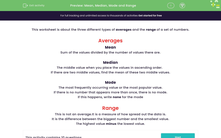# Find the Mean, Median, Mode and Range

In this worksheet, students will find the mean, median, mode and range of the given sets of numbers.Key stage:  KS 3

Curriculum topic:   Statistics

Curriculum subtopic:   Understand Variables, Representation, Measures and Spread

Popular topics:   Numbers worksheets

Difficulty level:#### Worksheet Overview

This activity is about the three different types of averages and the range of a set of numbers.

The three sets of averages are the mean, the median and the mode.

The mean

This is the sum of all the values, divided by the number of values that there are.

For example, here are the ages of a group of people who visited a gym one morning:

23, 28, 19, 25, 23, 31, 24

To find the mean age, we add up all the ages and divide by the number of ages, which is 7.

23 + 28 + 19 + 25 + 23 + 31 + 24 = 173

173 ÷ 7 = 24.7

The mean age of the gym members that morning is 44.7 to one decimal placeThe median

This is the middle value when you place the values in ascending order.

If there are two middle values, find the mean of these two middle values.

For example, if we take the same set of values from above, we can find the median age of gym members.

Put the ages in order first: 19, 23, 23, 24, 25, 28, 31

The middle value is 24, so this is the median age.

The mode

This is the most frequently occurring value or the most popular value.

It is possible to have more than one modal value.

If there is no number that appears more than once, there is no mode.

If this happens, write none for the mode.

For example, in the set of ages given above, the age 23 appears more often than any others. This means that the mode is 23.

The range

This is not an average. It is a measure of how spread out the data is.

It is the difference between the biggest number and the smallest value.

The highest value minus the lowest value.

In the set of ages above, the highest age is 31 and the lowest is 19

31 - 19 = 12 so the range is 12.

Let's have a go at some questions now.

### What is EdPlace?

We're your National Curriculum aligned online education content provider helping each child succeed in English, maths and science from year 1 to GCSE. With an EdPlace account you’ll be able to track and measure progress, helping each child achieve their best. We build confidence and attainment by personalising each child’s learning at a level that suits them.

Get started